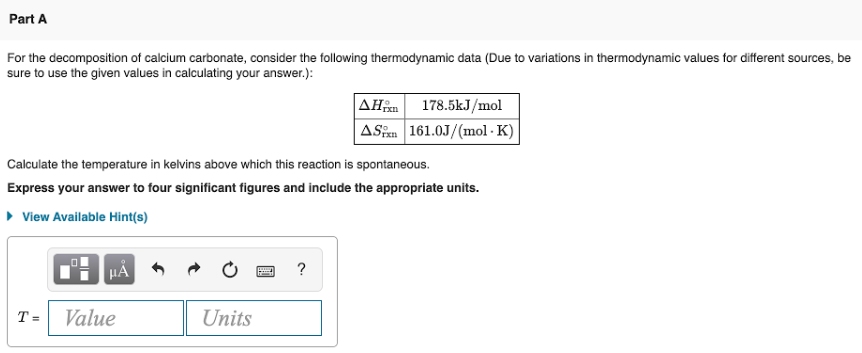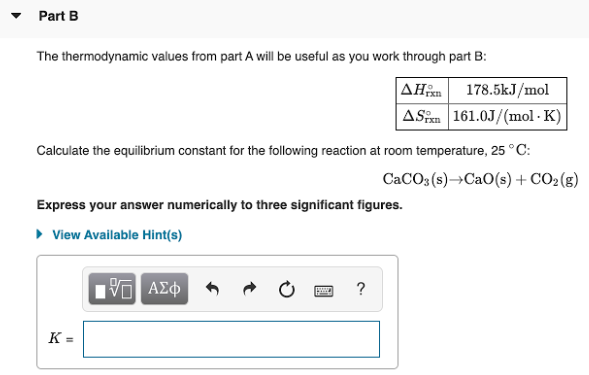# For the decomposition of calcium carbonate, consider the following thermodynamic data ( Due to variations in thermodynamic values for different sources, be sure to use the given values in calculating your answer.): ΔH°rxn 178.5kJ/mol ΔS°rxn 161.0J/(mol.K) Calculate the temperature in kelvins above which this reaction is spontaneous. Express your answer to four significant figures and include the appropriate units. The thermodynamic values from part A will be useful as you work through part B: ΔH°rxn 178.5kJ/mol ΔS°rxn 161.0J/(mol.K) Calculate the equilibrium constant for the following reaction at room temperature, 25°C: CaCO3(s) → CaO(s) + CO2(g) Express your answer numerically to three significant figures.